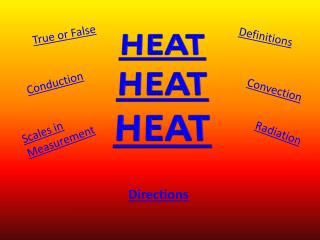DownloadDownload PresentationHEAT HEAT HEAT

# HEAT HEAT HEAT

Télécharger la présentation## HEAT HEAT HEAT

- - - - - - - - - - - - - - - - - - - - - - - - - - - E N D - - - - - - - - - - - - - - - - - - - - - - - - - - -
##### Presentation Transcript

1. HEAT HEATHEAT True or False Definitions Conduction Convection Scales in Measurement Radiation Directions

2. Directions • Choose a subject – True/False, Conduction, Insulator, Definitions, Convection, Radiation • Click on each question, and then carefully answer each. • If you get a question correct, move onto to the next question. • If you get a question incorrect, try again until you get it correct. HEAT

3. True or False • Question 1 • Question 2 • Question 3 • Question 4 HEAT

4. True or False • Temperature is the same as heat. True False HEAT

5. True or False • Heat can change the size of matter. (solids, liquids, and gases) TrueFalse HEAT

6. True or False • The amount of heat in matter depends on how fast the particles are moving and the amount of matter present. TrueFalse HEAT

7. True or False Water’s boiling point is 50°C and 120°F. TrueFalse HEAT

8. Conduction • Question 1 • Question 2 HEAT

9. Conduction Which kind of matter can heat move through most easily by conduction? AirMetalWater HEAT

10. Conduction The movement of heat through matter as particles __________ each other. LeaveBump HEAT

11. Scales in Measurement • Question 1 • Question 2 HEAT

12. Scales in Measurement What is freezing point of water? 24° F, 5° C32° F, 0° C HEAT

13. Scales in Measurement What is the boiling point of water? 212° F, 100° C57° F, 42° C HEAT

14. Definitions • Question 1 • Question 2 • Question 3 HEAT

15. Definition Heat is a form of energy that moves from ___________. Side to sideOne place to another HEAT

16. Definitions A thermometer is a tool used to _________ how hot or cold something is. MeasureMagnify HEAT

17. Definitions An insulator is any type of material that ______ allow heat to move easily. DoesDoes not HEAT

18. Convection • Question 1 • Question 2 HEAT

19. Convection Which kind of matter can heat move through most easily by convection? LiquidsLiquids and Gases HEAT

20. Convection The movement of heat through liquids and gases by ________. CurrentsWaves

21. Radiation • Question 1 • Question 2 HEAT

22. Radiation Which kind of matter can heat move through most easily by radiation? GasesEmpty space HEAT